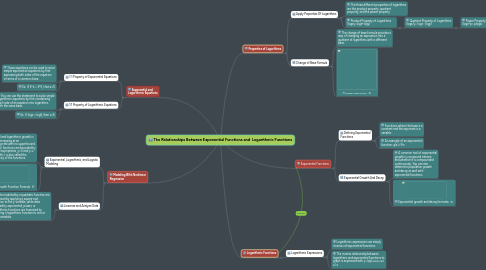# The Relationships Between Exponential Functions and Logarithmic FunctionsKASSIE KELEMEN
Get Started. It's FreeThe Relationships Between Exponential Functions and Logarithmic Functions## 1. Logarithmic Functions

### 1.1. Logarithmic Expressions

1.1.1. Logarithmic expressions are simply inverses of exponential functions.

1.1.2. The inverse relationship between logarithmic and exponential functions to graph is expressed with y=log(*subscript b*) x

## 2. Modeling With Nonlinear Regression

### 2.1. Exponential, Logarithmic, and Logistic Modeling

2.1.1. Exponential and logarithmic growth is unrestricted, increasing at an ever-increasing rate with no upper bound. Logistic growth functions are bounded by two horizontal asymptotes, y=0 and y=c. The limit growth c is also called the carrying capacity of the functions.

2.1.2. Logistic Growth Function Formula

### 2.2. Linearize and Analyze Data

2.2.1. Data modeled by a quadratic function are linearized by applying a square root function to the y-variable, while data molded by exponential, power, or logarithmic functions are linearized by applying a logarithmic function to one or both variables.

## 3. Exponential Functions

### 3.1. Defining Exponential Functions

3.1.1. Functions where the base is a constant and the exponent is a variable.

3.1.2. An example of an exponential function: g(x)=3^x

### 3.2. Exponential Growth And Decay

3.2.1. A common tool of exponential growth is compound interest and whether it is compounded continuously. You can also determine population growth and decay as well with exponential functions.

3.2.2. Exponential growth and decay formulas

## 4. Properties of Logarithms

### 4.1. Apply Properties Of Logarithms

4.1.1. The three different properties of logarithms are the product property, quotient property, and the power property.

4.1.2. Product Property of Logarithms (logxy=logx+logy)

4.1.2.1. Quotient Property of Logarithms (logx/y= logx - logy)

4.1.2.1.1. Power Property of Logarithms (logx^p= plogx)

### 4.2. Change of Base Formula

4.2.1. The change of base formula provides a way of changing an expression into a quotient of logarithms with a different base.

4.2.2. Change of Base Formula

## 5. Exponential and Logarithmic Equations

### 5.1. 1:1 Property of Exponential Equations

5.1.1. These equations can be used to solve simple exponential equations by first expressing both sides of the equation in terms of a common base.

5.1.2. Ex. If 3^x = 3^5, then x=5

### 5.2. 1:1 Property of Logarithmic Equations

5.2.1. You can use the statement to solve simple logarithmic equations by first condensing each side of an equation into logarithms with the same base.

5.2.2. Ex. If logx = log6, then x=6